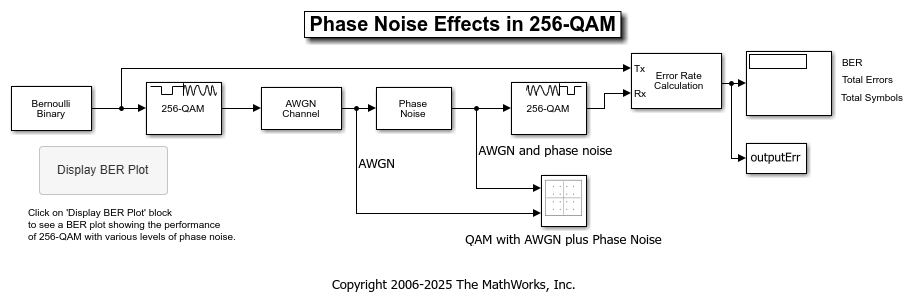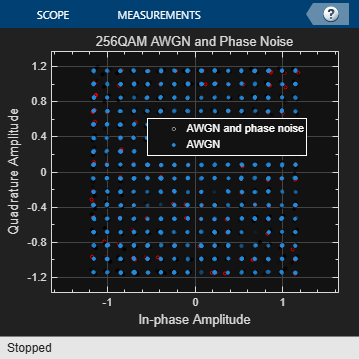This example shows you the basic functionality of a communications system that models quadrature amplitude modulation (QAM), additive white Gaussian noise (AWGN) channel, and phase noise using Simulink®. The model displays constellation diagrams of the 256-QAM signal and performs error rate computations.### Overview

The `cm_commphasenoise` model, simulates the effect of AWGN and phase noise on a 256-QAM signal. The Simulink model is a graphical representation for a mathematical model of a communications system that generates a random signal, modulates it using QAM, adds AWGN and phase noise to the signal, and demodulates the signal. The model also contains blocks to display the bit error rate and constellation diagrams of the modulated signal.

• The Bernoulli Binary Generator block generates a signal consisting of a sequence of 8-bit binary values in the range [0, 255].

• The Rectangular QAM Modulator Baseband block modulates the signal using baseband 256-ary QAM.

• The AWGN Channel block models a noisy channel by adding white Gaussian noise to the modulated signal.

• The Phase Noise block introduces noise in the angle of its complex input signal.

• The Rectangular QAM Demodulator Baseband block demodulates the signal.

• The Constellation Diagram block displays constellation diagrams of the signal with AWGN and phase noise added.

• The Error Rate Calculation block counts bits that differ between the received signal and transmitted signal.

• The To Workspace block, labeled `outputErr`, outputs the results to the workspace for use when plotting the results. The Display BER Plot block opens a bit error rate (BER) plot showing the Eb/N0 performance curves for 256-QAM transmission and reception at various levels of phase noise.

### Digital Modulation

The model simulates QAM, which is a method for converting a digital signal to a complex signal. The model modulates the signal onto a sequence of complex numbers that lie on a lattice of points in the complex plane, known as the constellation of the signal. A plot of these points is called a scatterplot or constellation diagram of the signal.

The constellation diagram shown here displays the baseband 256-ary QAM with AWGN added and with AWGN and phase noise added. The points in the constellation diagram do not lie exactly on the constellation shown in the figure because of the added noise. Phase noise alters the angle of the complex modulated signal, causing a radial displacement of constellation points.### Run the Simulation

The default model configuration has the run duration set to `inf`. The Error Rate Calculation block is configured to run until 100 errors occur. To stop the simulation before 100 errors occur, click Stop on the Simulation tab.

### Display the Error Rate

The Display block displays the number of errors introduced by the AWGN channel and phase noise. When you run the simulation, three small boxes appear in the block, displaying the vector output from the Error Rate Calculation block.

• The first entry is the BER.

• The second entry is the total number of errors.

• The third entry is the total number of comparisons made.

Display a Phase Noise Plot

To display a figure that plots simulation results of BER versus Eb/N0 curves for a range of phase noise settings, double-click the Display BER Plot block in the model.

### Further Exploration

You can control the way a Simulink block functions by setting its parameters. To view or change simulation parameters, double-click a block to open its block mask.

To change the amount of phase noise, open the Phase Noise block mask and enter a new value for the Phase noise level (dBc/Hz) parameter. Click OK to apply the new setting.

To change the amount of noise, open the AWGN Channel block mask and enter a new value for the Eb/No (dB) parameter. Decreasing this parameter value increases the noise level. Click OK to apply the new setting.

Reducing the phase noise and increasing the Eb/N0 removes noise from the model. Since the model is configured to run until 100 errors occur, running the simulation with little noise in the model results in a long simulation run time. To limit the maximum simulation run time, you can reduce the run duration from `inf` to a small value, such as `10`.

To produce new results, run the simulation using the modified settings.

Alternatively, you can enter a variable name in a parameter. Then at the MATLAB® command line set the value for that variable in the workspace. Setting parameters in the Command Window can be convenient if you need to run multiple simulations with different parameter values.

You can also use callback functions to configure your simulation. The default setting for several parameters in this model are set using the `PreLoadFcn` callback function. To access the callback functions, select Model Settings > Model Properties on the Modeling tab. In the Model Properties dialog, select the Callbacks tab. For more information on model properties and callback functions, see Model Callbacks (Simulink).

Plot BER at Different Noise Levels

The `plot_256qam_ber_curves.m` MATLAB® program file generated this BER plot by running multiple simulations with different values for the Phase noise level (dBc/Hz) and Eb/No (dB) parameters. Each curve is a plot of BER as a function of signal to noise ratio for a fixed amount of phase noise. For each plotted BER point, the simulation stopped when 1000 bit errors were reached or 1e8 bits were compared. Results vary from run to run due to the random nature of the input signal and simulation impairments.